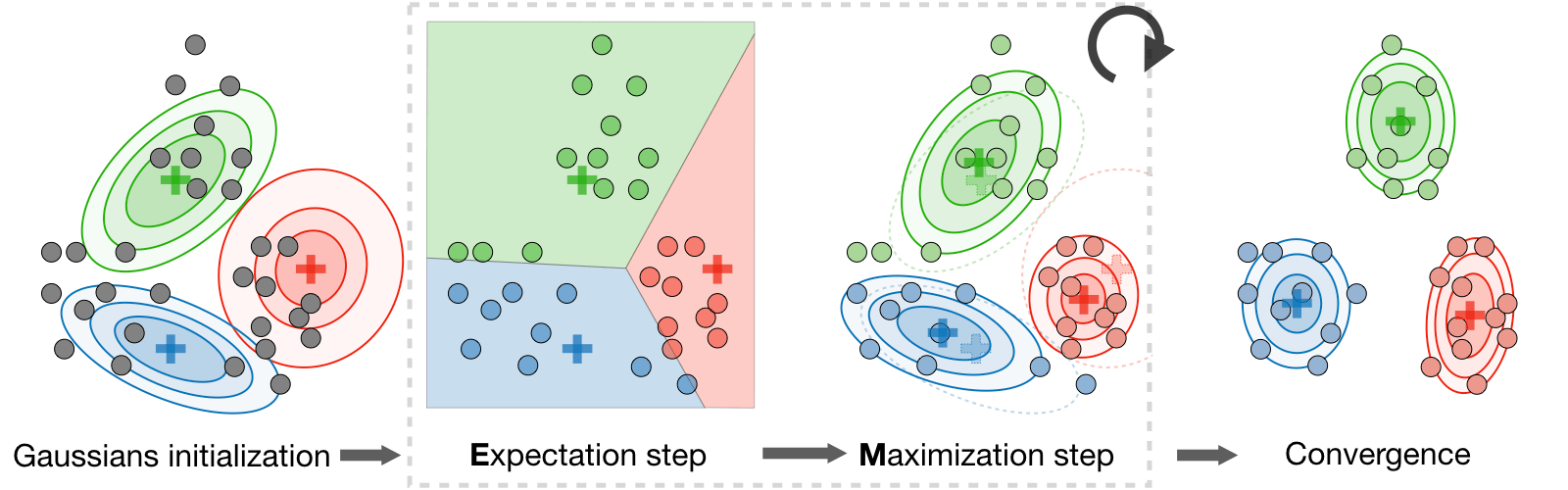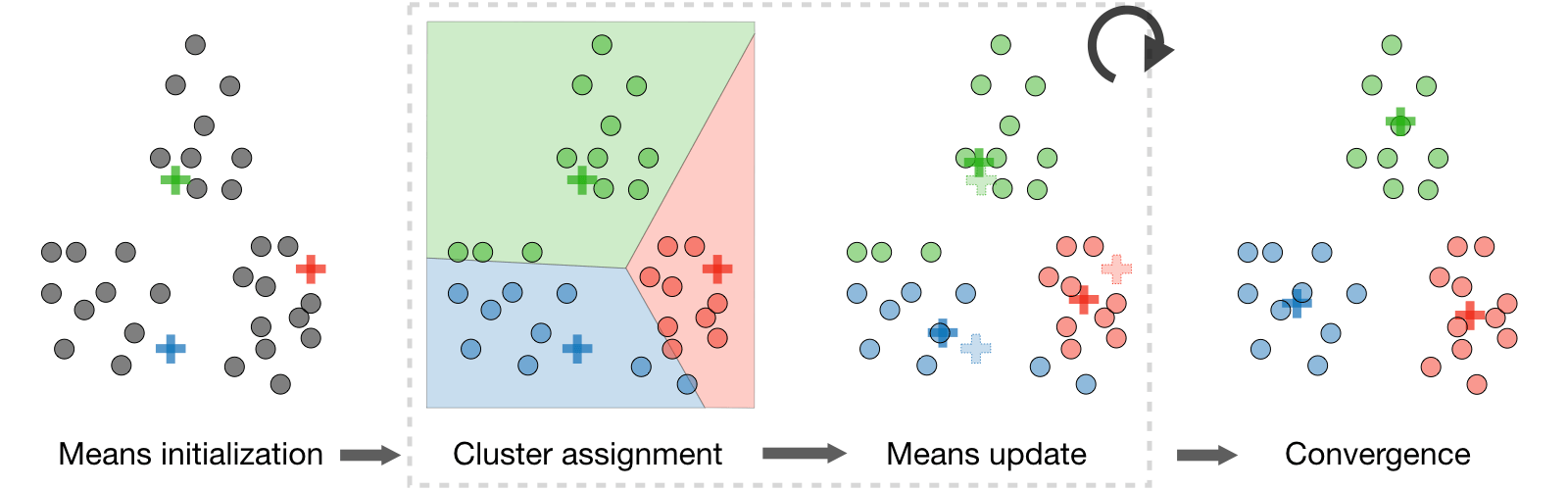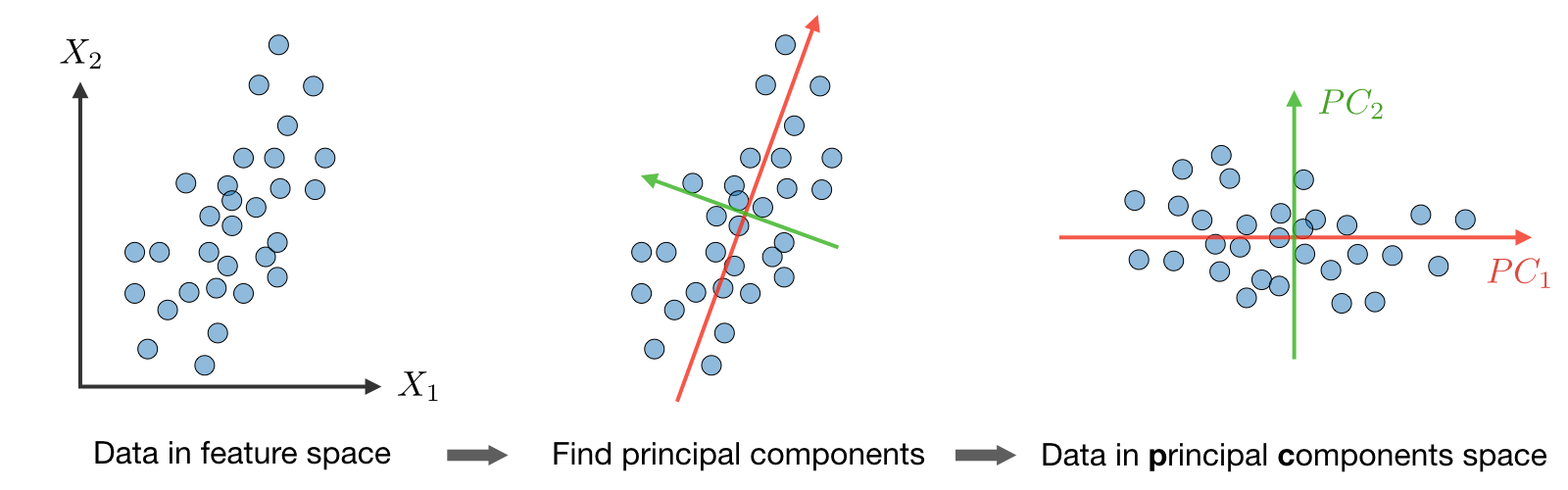cheatsheet最后更新于：2020年6月30日

# Unsupervised Learning cheatsheet

## Introduction to Unsupervised Learning

• Motivation: The goal of unsupervised learning is to find hidden patterns in unlabeled data $\{x^{(1)},...,x^{(m)}\}$.
• Jensen's inequality: Let $f$ be a convex function and $X$ a random variable. We have the following inequality:

$\boxed{E[f(X)]\geqslant f(E[X])}$

## Clustering

### Expectation-Maximization

• Latent variables: Latent variables are hidden/unobserved variables that make estimation problems difficult, and are often denoted $z$. Here are the most common settings where there are latent variables:
Setting Mixture of $k$ Gaussians Factor analysis
Latent variable $z$ $\textrm{Multinomial}(\phi)$ $\mathcal{N}(0,I)$
$x|z$ $\mathcal{N}(\mu_j,\Sigma_j)$ $\mu_j\in\mathbb{R}^n, \phi\in\mathbb{R}^k$
Comments $\mathcal{N}(\mu+\Lambda z,\psi)$ $\mu_j\in\mathbb{R}^n$
• Algorithm: The Expectation-Maximization (EM) algorithm gives an efficient method at estimating the parameter $\theta$ through maximum likelihood estimation by repeatedly constructing a lower-bound on the likelihood (E-step) and optimizing that lower bound (M-step) as follows:

• E-step: Evaluate the posterior probability $Q_{i}(z^{(i)})$ that each data point $x^{(i)}$ came from a particular cluster $z^{(i)}$ as follows:

$\boxed{Q_i(z^{(i)})=P(z^{(i)}|x^{(i)};\theta)}$

• M-step: Use the posterior probabilities $Q_i(z^{(i)})$ as cluster specific weights on data points $x^{(i)}$ to separately re-estimate each cluster model as follows:

$\boxed{\theta_i=\underset{\theta}{\textrm{argmax }}\sum_i\int_{z^{(i)}}Q_i(z^{(i)})\log\left(\frac{P(x^{(i)},z^{(i)};\theta)}{Q_i(z^{(i)})}\right)dz^{(i)}}$### $k$-means clustering

We note $c^{(i)}$ the cluster of data point $i$ and $\mu_j$ the center of cluster $j$.

• Algorithm: After randomly initializing the cluster centroids $\mu_1,\mu_2,...,\mu_k\in\mathbb{R}^n$, the $k$-means algorithm repeats the following step until convergence:

$\boxed{c^{(i)}=\underset{j}{\textrm{arg min}}\|x^{(i)}-\mu_j\|^2}\quad\textrm{and}\quad\boxed{\mu_j=\frac{\displaystyle\sum_{i=1}^m1_{\{c^{(i)}=j\}}x^{(i)}}{\displaystyle\sum_{i=1}^m1_{\{c^{(i)}=j\}}}}$• Distortion function: In order to see if the algorithm converges, we look at the distortion function defined as follows:

$\boxed{J(c,\mu)=\sum_{i=1}^m\|x^{(i)}-\mu_{c^{(i)}}\|^2}$

### Hierarchical clustering

• Algorithm: It is a clustering algorithm with an agglomerative hierarchical approach that build nested clusters in a successive manner.
• Types: There are different sorts of hierarchical clustering algorithms that aims at optimizing different objective functions, which is summed up in the table below:
Minimize within cluster distance Minimize average distance between cluster pairs Minimize maximum distance of between cluster pairs

### Clustering assessment metrics

In an unsupervised learning setting, it is often hard to assess the performance of a model since we don't have the ground truth labels as was the case in the supervised learning setting.

• Silhouette coefficient: By noting $a$ and $b$ the mean distance between a sample and all other points in the same class, and between a sample and all other points in the next nearest cluster, the silhouette coefficient $s$ for a single sample is defined as follows:

$\boxed{s=\frac{b-a}{\max(a,b)}}$

• Calinski-Harabaz index: By noting $k$ the number of clusters, $B_k$ and $W_k$ the between and within-clustering dispersion matrices respectively defined as

\begin{align} B_k&=\sum_{j=1}^kn_{c^{(i)}}(\mu_{c^{(i)}}-\mu)(\mu_{c^{(i)}}-\mu)^T,\\ W_k&=\sum_{i=1}^m(x^{(i)}-\mu_{c^{(i)}})(x^{(i)}-\mu_{c^{(i)}})^T \end{align}

the Calinski-Harabaz index $s(k)$ indicates how well a clustering model defines its clusters, such that the higher the score, the more dense and well separated the clusters are. It is defined as follows: $\boxed{s(k)=\frac{\textrm{Tr}(B_k)}{\textrm{Tr}(W_k)}\times\frac{N-k}{k-1}}$

## Dimension reduction

### Principal component analysis

It is a dimension reduction technique that finds the variance maximizing directions onto which to project the data.

• Eigenvalue, eigenvector: Given a matrix $A\in\mathbb{R}^{n\times n}$, $\lambda$ is said to be an eigenvalue of $A$ if there exists a vector $z\in\mathbb{R}^n\backslash\{0\}$, called eigenvector, such that we have:

$\boxed{Az=\lambda z}$

• Spectral theorem: Let $A\in\mathbb{R}^{n\times n}$. If $A$ is symmetric, then $A$ is diagonalizable by a real orthogonal matrix $U\in\mathbb{R}^{n\times n}$. By noting $\Lambda=\textrm{diag}(\lambda_1,...,\lambda_n)$, we have:

$\boxed{\exists\Lambda\textrm{ diagonal},\quad A=U\Lambda U^T}$

Remark: the eigenvector associated with the largest eigenvalue is called principal eigenvector of matrix $A$.

• Algorithm: The Principal Component Analysis (PCA) procedure is a dimension reduction technique that projects the data on $k$ dimensions by maximizing the variance of the data as follows:

• Step 1: Normalize the data to have a mean of 0 and standard deviation of 1. \boxed{ \begin{align} x_j^{(i)}&\leftarrow\frac{x_j^{(i)}-\mu_j}{\sigma_j}\\ \mu_j &= \frac{1}{m}\sum_{i=1}^mx_j^{(i)}\\ \sigma_j^2&=\frac{1}{m}\sum_{i=1}^m(x_j^{(i)}-\mu_j)^2 \end{align} }

• Step 2: Compute $\displaystyle\Sigma=\frac{1}{m}\sum_{i=1}^mx^{(i)}{x^{(i)}}^T\in\mathbb{R}^{n\times n}$, which is symmetric with real eigenvalues.

• Step 3: Compute $u_1, ..., u_k\in\mathbb{R}^n$ the $k$ orthogonal principal eigenvectors of $\Sigma$, i.e. the orthogonal eigenvectors of the $k$ largest eigenvalues.

• Step 4: Project the data on $\textrm{span}_\mathbb{R}(u_1,...,u_k)$.

This procedure maximizes the variance among all $k$-dimensional spaces.### Independent component analysis

It is a technique meant to find the underlying generating sources.

• Assumptions: We assume that our data $x$ has been generated by the $n$-dimensional source vector $s=(s_1,...,s_n)$, where $s_i$ are independent random variables, via a mixing and non-singular matrix $A$ as follows:

$\boxed{x=As}$

The goal is to find the unmixing matrix $W=A^{-1}$.

• Bell and Sejnowski ICA algorithmThis algorithm finds the unmixing matrix $W$ by following the steps below:

• Write the probability of $x=As=W^{-1}s$ as: $p(x)=\prod_{i=1}^np_s(w_i^Tx)\cdot|W|$

• Write the log likelihood given our training data $\{x^{(i)}, i\in[\![1,m]\!]\}$ and by noting $g$ the sigmoid function as: $l(W)=\sum_{i=1}^m\left(\sum_{j=1}^n\log\Big(g'(w_j^Tx^{(i)})\Big)+\log|W|\right)$

Therefore, the stochastic gradient ascent learning rule is such that for each training example $x^{(i)}$, we update $W$ as follows: $\boxed{W\longleftarrow W+\alpha\left(\begin{pmatrix}1-2g(w_1^Tx^{(i)})\\1-2g(w_2^Tx^{(i)})\\\vdots\\1-2g(w_n^Tx^{(i)})\end{pmatrix}{x^{(i)}}^T+(W^T)^{-1}\right)}$